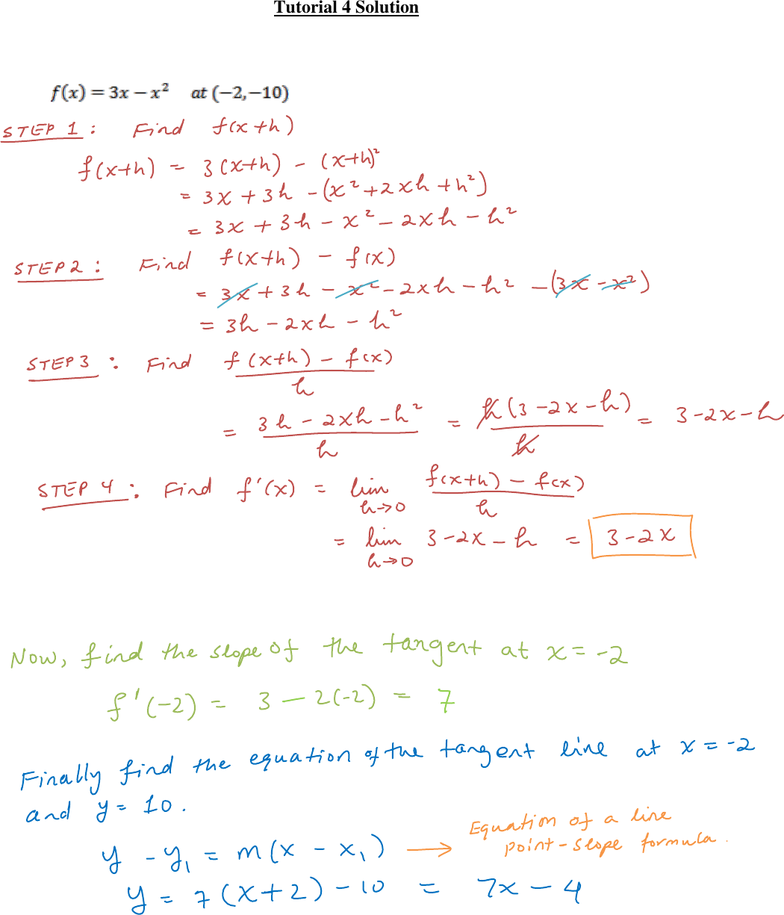# Tutorial_4_Solution.pdf

36 views7 pages
School
Department
Course
Page:
of 7Tutorial 4 Solution
1. Find the slope of the tangent line to the graph of the function at the given point, and
determine an equation of the tangent line (Use the basic definition of derivatives).
2. Find the derivative of each function.
a.
b.
c.
d.